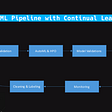# Standard k-mean Algorithm implemented in python

link to know — what is standard k-mean algorithm

https://en.wikipedia.org/wiki/K-means_clustering#Standard_algorithm

Here is 1st a Point class –

`from math import sqrtclass Point(object):    def __init__(self, x, y):       self.x=int(x)       self.y=int(y)       def distance(self, other):       return sqrt(( self.x - other.x)**2 +( self.y - other.y)**2)    def __add__(self,p):       localx = self.x + p.x       localy = self.y + p.y       return Point(localx, localy)    def __truediv__(self, p):       self.x = self.x / p       self.y = self.y / p       return self   def __repr__(self):       return str(self.x) +' '+ str(self.y)`

Here is Cluster class for clustering based on points near to center point.

`class Cluster(object):    def __init__(self, x, y):        self.center = Point(x, y)        self.points = []        def update(self):        temp=Point(0, 0)        #print(len(self.points))        count=0        for point in self.points:            count+=1            temp += point        print(len(self.points))        self.center =  temp/count        self.points = []    def add_point(self, point):        self.points.append(point)`

Here is Compute Class in which we did all stuff of calculating dist

`def compute_result(points):    points = [Point(*point) for point in points]    a = Cluster(1,0)    b = Cluster(-1,0)    a_old = []    for _ in range(10000): # max iterations        for point in points:            if point.distance(a.center) < point.distance(b.center):                # add the right point                a.add_point(point)            else:                # add the right point                b.add_point(point)        if a_old == a.points:            break        a_old = a.points        a.update()        b.update()    print(a.center.y)    return [(a.center.x, a.center.y), (b.center.x, b.center.y)]`

Check More on : https://codedatascience.com/

--

--

--

## More from Mando

Research Machine Learning Engineer

Love podcasts or audiobooks? Learn on the go with our new app.

## SQA Engineer to Dev?## SQL Database with Python## The Hidden Factor of Company Size: Why Your Project Team Should Be a Part of Something Bigger## Installation and usage of UniConvertor(Sk1 project): A file conversion tool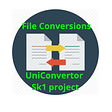## Ballerina REST API service with Cosmos DB connector — Part2## Lightning Node Performance: Testing TPS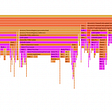## Control layout and styling in Build Annotations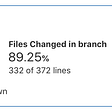## Automatic Flamegraphs for Benchmarks in Rust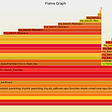## Mando

Research Machine Learning Engineer

## Applying Graph Theory concepts in basic data manipulation problems## Real-time classification with Deephaven and SciKit-Learn## Ultimate python DateParser with holidays, paydays and all the good stuff.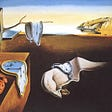## Explore how to apply continual learning to your machine learning models